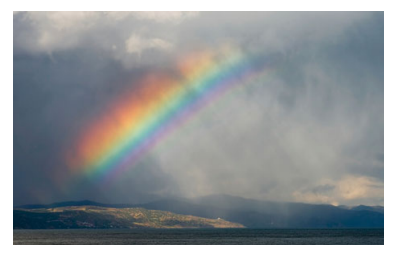×
Get Full Access to Chemistry: The Central Science - 12 Edition - Chapter 6 - Problem 5e
Get Full Access to Chemistry: The Central Science - 12 Edition - Chapter 6 - Problem 5e

×

# Visualizing ConceptsThe familiar phenomenon of a rainbowISBN: 9780321696724 27

## Solution for problem 5E Chapter 6

Chemistry: The Central Science | 12th Edition

• Textbook Solutions
• 2901 Step-by-step solutions solved by professors and subject experts
• Get 24/7 help from StudySoup virtual teaching assistantsChemistry: The Central Science | 12th Edition

4 5 1 372 Reviews
21
3
Problem 5E

The familiar phenomenon of a rainbow results from the diffraction of sunlight through raindrops. (a) Does the wavelength of light increase or decrease as we proceed outward from the innermost band of the rainbow? (b) Does the frequency of light increase or decrease as we proceed outward? (c) Suppose that instead of sunlight, the visible light from a hydrogen discharge tube (Figure 6.10) was used as the light source. What do you think the resulting “hydrogen discharge rainbow” would look like? [Section 6.3]Step-by-Step Solution:
Step 1 of 3

AY 101: Week 5 Lecture Notes: Feb. 14 & 16 Light I. What Is Light i. Light is energy, a wave, a particle, and sight. a. Light as Electromagnetic Waves a. Properties of light: i. Speed: 300,000 km/s ii. Wavelength: varies, but is defined as the spatial distance from peak to peak. b. The Electromagnetic Spectrum:  Must know the basic rays/waves and their order for exam 2! II. What Are Radio Waves i. Radio waves are long wavelengths of light, NOT sound! 1. Broadcasters encode audio signals in the amplitude

Step 2 of 3

Step 3 of 3

##### ISBN: 9780321696724

This textbook survival guide was created for the textbook: Chemistry: The Central Science, edition: 12. The full step-by-step solution to problem: 5E from chapter: 6 was answered by , our top Chemistry solution expert on 04/03/17, 07:58AM. The answer to “?The familiar phenomenon of a rainbow results from the diffraction of sunlight through raindrops. (a) Does the wavelength of light increase or decrease as we proceed outward from the innermost band of the rainbow? (b) Does the frequency of light increase or decrease as we proceed outward? (c) Suppose that instead of sunlight, the visible light from a hydrogen discharge tube (Figure 6.10) was used as the light source. What do you think the resulting “hydrogen discharge rainbow” would look like? [Section 6.3]” is broken down into a number of easy to follow steps, and 83 words. Chemistry: The Central Science was written by and is associated to the ISBN: 9780321696724. Since the solution to 5E from 6 chapter was answered, more than 599 students have viewed the full step-by-step answer. This full solution covers the following key subjects: light, hydrogen, rainbow, decrease, outward. This expansive textbook survival guide covers 49 chapters, and 5471 solutions.

Unlock Textbook Solution